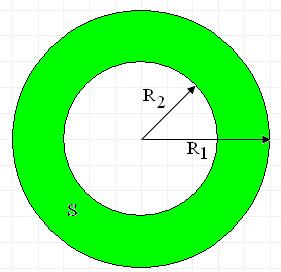Problems

# RingThe area of a ring and radius of an external circle are given. Find the radius of an internal circle.

#### Input

Two real numbers are given: the area of a ring and the radius of an external circle, which does not exceed 100.

#### Output

Print the radius of an internal circle with 2 digits after the decimal point.

Time limit 1 second
Memory limit 122.17 MiB
Input example #1
50.2655 5

Output example #1
3.00

Source SFE-2010 Variant 24